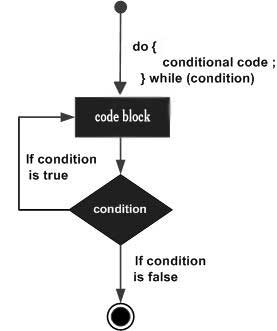# do while loop in java

A do...while loop is similar to a while loop, except that a do...while loop is guaranteed to execute at least one time.

## Syntax

Following is the syntax of a do...while loop −

```do {
// Statements
}while(Boolean_expression);
```

Notice that the Boolean expression appears at the end of the loop, so the statements in the loop execute once before the Boolean is tested.

If the Boolean expression is true, the control jumps back up to do statement, and the statements in the loop execute again. This process repeats until the Boolean expression is false.

## Flow Diagram## Example

```public class Test {

public static void main(String args[]) {
int x = 10;

do {
System.out.print("value of x : " + x );
x++;
System.out.print("\n");
}while( x < 20 );
}
}
```

This will produce the following result −

## Output

```value of x : 10
value of x : 11
value of x : 12
value of x : 13
value of x : 14
value of x : 15
value of x : 16
value of x : 17
value of x : 18
value of x : 19
```
java_loop_control.htm